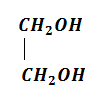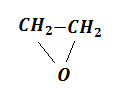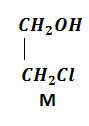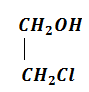In a reaction,

${\mathrm{CH}}_{2}={\mathrm{CH}}_{2}\underset{\mathrm{acid}}{\overset{\mathrm{Hypochlorous}}{\to }}$M$\stackrel{\mathrm{R}}{\to }$M=molecules, R=reagent. M and R are

(a) ${\mathrm{CH}}_{3}{\mathrm{CH}}_{2}\mathrm{Cl}$ and NaOH

(b)  and aq. ${\mathrm{NaHCO}}_{3}$

(c) ${\mathrm{CH}}_{3}{\mathrm{CH}}_{2}\mathrm{OH}$ and HCl

(d)and heat

Concept Videos :-

#1 | Preparation of Alcohols
#2 | Preparation of Alcohols: II
#3 | Preparation of Alcohols: III
#4 | Properties of Alcohols & Phenols (Comparative)
#5 | Chemical Properties of Alcohols
#7 | Preparation & Properties of Dihydric Alcohols
#8 | Reactions of Dihydric Alcohol
#9 | Preparation & Properties of Trihydric Alcohols

Concept Questions :-

Alcohols: Preparation, Physical and Chemical Properties

(b) ${\mathrm{CH}}_{2}={\mathrm{CH}}_{2}\stackrel{\mathrm{HOCl}}{\to }$$\underset{{\mathrm{NaHCO}}_{3}\left(\mathrm{aq}\right)}{\overset{\mathrm{R}}{\to }}$So,M isand R is ${\mathrm{NaHCO}}_{3}$.

Difficulty Level:

• 21%
• 53%
• 10%
• 18%